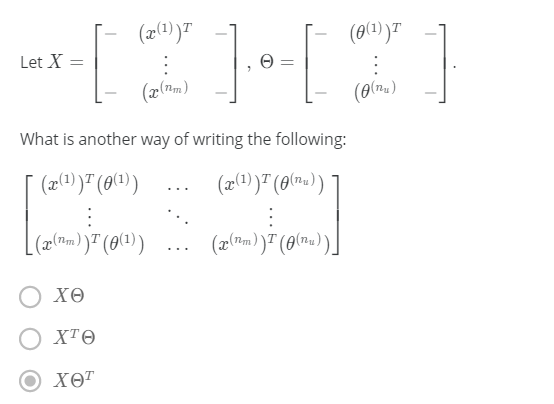# Recommender Systems - Class Review

Last updated on：a year ago

Recommender systems are powerful tools to effectively predict people’s taste. Thus, it has huge business value for advertisers.

# Notation

eg. predicting movies rating

$n_u$ No. of users

$n_m$ no. movies

$r(i,j) = 1$ if user j has rated movie i

$y^{(i,j)}$ rating given by user j to movie i (defined only if $r(i,j) = 1$)

$i:r(i,j) = 1$ means: for each each j, all i when $r(i,j) = 1$.

$j:r(i,j) = 1$ means: for each each i, all j when $r(i,j) = 1$.

$(i,j):r(i,j) = 1$ means: all (i,j) when $r(i,j) = 1$.

# Content-based recommendations

Distract movie’s feature vectors

$\theta^{(j)}$ parameter vector for user j

$x^{(i)}$ feature vector for movie i

For user j, movie i, predicted rating: $( \theta^{(j)} )^T x^{(i)}$.

$m^{(j)}$ No. of movies rated by user j

## To learn $\theta$

Given $x^{(1)}, x^{(2)}, …, x^{(n_m)}$ and movie ratings, can estimate $\theta^{(1)}, \theta^{(2)}, …, \theta^{(n_u)}$.

To learn $\theta^{(j)}$

$$\min_{\theta^{(j)}}\frac{1}{2} \sum_{i:r(i,j) = 1} (( \theta^{(j)}) ^T x^{(i)} - y^{(i,j)}) ^2 + \frac{\lambda}{2} \sum^n_{k=1} ( \theta^{j}_k) ^2$$

To learn $\theta^{(1)}, \theta^{(2)}, …, \theta^{(n_u)}$

$$\min_{\theta^{(1)}, …, \theta^{(n_u)}} \frac{1}{2} \sum^{n_u}_{j=1} \sum_{i:r(i,j) = 1} (( \theta^{(j)}) ^T x^{(i)} - y^{(i,j)}) ^2 + \frac{\lambda}{2} \sum^{n_u}_{j=1} \sum^n_{k=1} ( \theta^{j}_k) ^2$$

When $k = 0$,

$$\theta_k^{(j)}:= \theta_k^{(j)} - \alpha \sum_{i:r(i,j)=1} (( \theta^{(j)}) ^T x^{(i)} - y^{(i,j)}) x_k^{(i)}$$

When $k = 1$,

$$\theta_k^{(j)}:= \theta_k^{(j)} - \alpha ( \sum_{i:r(i,j)=1} (( \theta^{(j)}) ^T x^{(i)} - y^{(i,j)}) x_k^{(i)} + \lambda \theta_k^{(j)})$$

## To learn $x^{(i)}$

Given $\theta^{(1)}, \theta^{(2)}, …, \theta^{(n_u)}$, can estimate $x^{(1)}, x^{(2)}, …, x^{(n_m)}$.

To learn $x^{(i)}$

$$\min_{x^{(j)}}\frac{1}{2} \sum_{j:r(i,j) = 1} (( \theta^{(j)}) ^T x^{(i)} - y^{(i,j)}) ^2 + \frac{\lambda}{2} \sum^n_{k=1} ( x^{i}_k) ^2$$

To learn $x^{(1)}, x^{(2)}, …, x^{(n_m)}$

$$\min_{x^{(1)}, …, x^{(n_m)}} \frac{1}{2} \sum^{n_m}_{i=1} \sum_{j:r(i,j) = 1} (( \theta^{(j)}) ^T x^{(i)} - y^{(i,j)}) ^2 + \frac{\lambda}{2} \sum^{n_m}_{i=1} \sum^n_{k=1} ( x^{j}_k) ^2$$

When $k = 0$,

$$x_k^{(j)}:= x_k^{(i)} - \alpha \sum_{j:r(i,j)=1} (( \theta^{(j)}) ^T x^{(i)} - y^{(i,j)}) \theta_k^{(j)}$$

When $k = 1$,

$$x_k^{(j)}:= x_k^{(i)} - \alpha \sum_{j:r(i,j)=1} (( \theta^{(j)}) ^T x^{(i)} - y^{(i,j)}) \theta_k^{(j)} + \lambda x_k^{(i)})$$

## Collaborating filtering algorithm

Minimizing $x^{(1)}, x^{(2)}, …, x^{(n_m)}$ and $\theta^{(1)}, \theta^{(2)}, …, \theta^{(n_u)}$ simultaneously:

$$J(x^{(1)}, …, x^{(n_m)}, \theta^{(1)}, …, \theta^{(n_u)}) = \frac{1}{2} \sum_{(i,j):r(i,j) = 1} ((\theta^{(j)} )^T x^{(i)} - y^{(i,j)} )^2 + \frac{\lambda}{2} \sum^{n_u}_{j=1} \sum^n_{k=1} ( \theta^{j}_k) ^2 + \frac{\lambda}{2} \sum^{n_m}_{i=1} \sum^n_{k=1} ( x^{j}_k) ^2$$

$$\min_{x^{(1)}, …, x^{(n_m)}, \theta^{(1)}, …, \theta^{(n_u)}} J(x^{(1)}, …, x^{(n_m)}, \theta^{(1)}, …, \theta^{(n_u)})$$

Gradient descent is the same to the previous content.

## Steps

• Initialize $x^{(1)}, …, x^{(m)}, \theta^{(1)}, …,\theta^{(m_u)}$ to random values
• Minimize $J(x^{(1)}, …, x^{(m)}, \theta^{(1)}, …,\theta^{(m_u)})$

Descent (or an advanced optimization algorithm) eg. for every $j = 1, …, n_u, i = 1, …, n_m$

• For user with parameters $\theta$ and a movie with (learned) features x, predict a star rating of $\theta^Tx$

# Implementing detail: mean normalization

We talked about mean normalization. However, unlike some other applications of feature scaling, we did not scale the movie ratings by dividing by the range (max – min value). This is because:

All the movie ratings are already comparable (e.g., 0 to 5 stars), so they are already on similar scales.

# Class exercises

## Within classes

Q1: Vectorization: low-rank matrix factorization## Homework

Q2: In which of the following situations will a collaborative filtering system be the most appropriate learning algorithm (compared to linear or logistic regression)?
You’ve written a piece of software that has downloaded news articles from many news websites. in /– your system, you also keep track of which articles you personally like vs. dislike, and the system also stores away features of these articles (e.g., word counts, name of author]. Using this information, you want to build a system to try to find additional new articles that you personally will like.

- [x] You manage an online bookstore and you have the book ratings from many users. For each user, you want to recommend other books she will enjoy, based on her own ratings and the ratings of other users.
- [x] You run an online news aggregator, and for every user, you know some subset of articles that the user likes and some different subset that the user dislikes. You’d want to use this to find other articles that the user likes.

You manage an online bookstore and you have the book ratings from many users. You want to learn to predict the expected sales volume {number of books sold] as a function of the average rating of a book.

Q4: Which of the following are true of collaborative filtering systems? Check all that apply.
Suppose you are writing a recommender system to predict a user’s book preferences. In order to build such a system, you need the user to rate all the other books in your training set.

- [x] For collaborative filtering, it is possible to use one of the advanced optimization algorithms (L-BFGS/conjugate gradient, etc.) to solve for both the $x^{(i)}$’s and $\theta^{(j)}$’s simultaneously.

For collaborative filtering, the optimization algorithm you should use is gradient descent. In particular, you cannot use more advanced optimization algorithms {L-BFGS/conjugate gradient, etc.) for collaborative filtering, since you have to solve for both the $x^{(i)}$’s and $\theta^{(j)}$’s simultaneously.

- [x] Even if each user has rated only a small fraction of all of your products (so $r(i,j) = 0$ for the vast majority of $(i,j)$ pairs), you can still build a recommender system by using collaborative filtering.

# Reference

 Andrew NG, Machine Learning